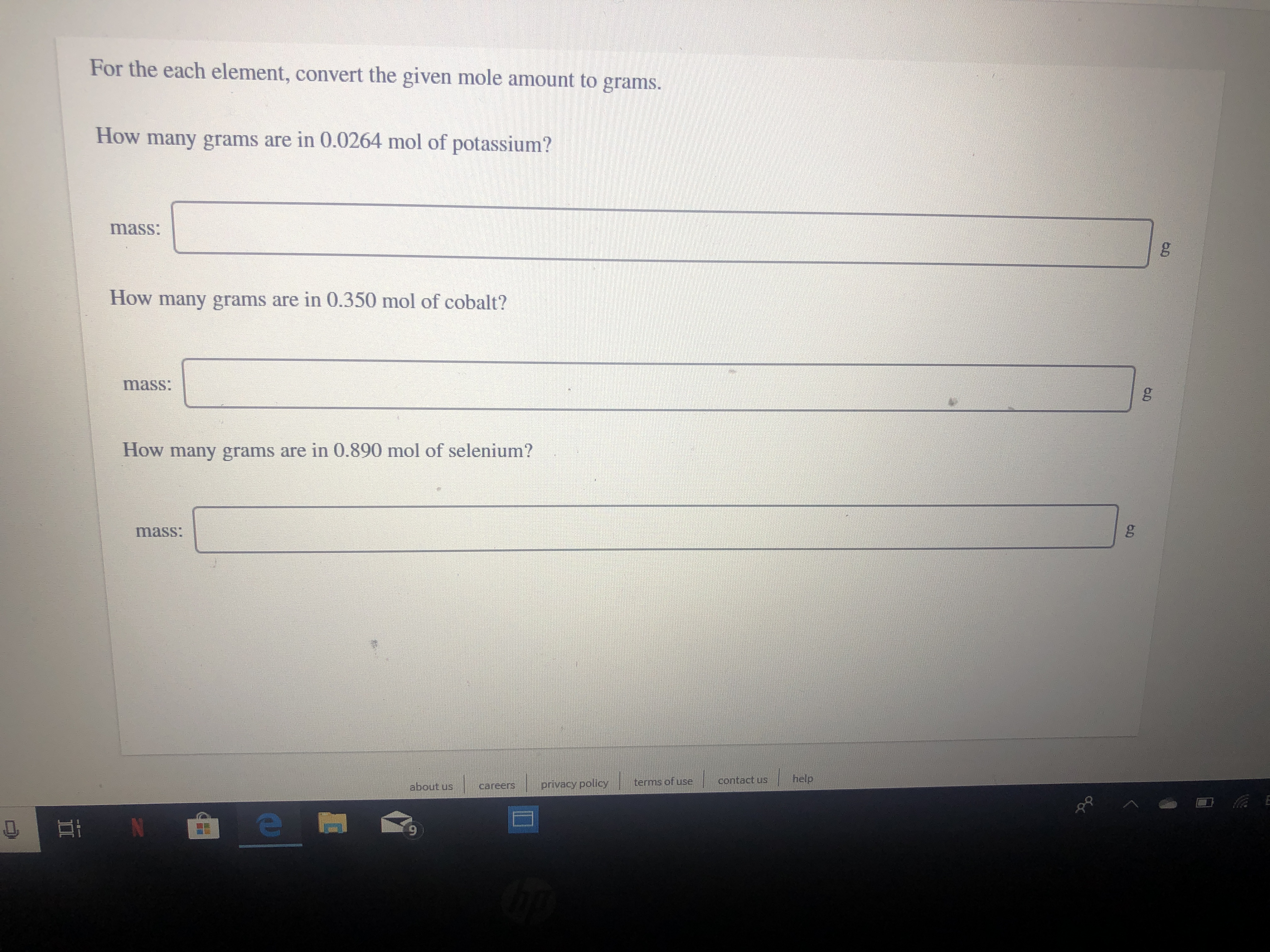For the each element, convert the given mole amount to grams.How many grams are in 0.0264 mol of potassium?mass:How many grams are in 0.350 mol of cobalt?mass:How many grams are in 0.890 mol of selenium?mass:helpcontact usterms of useprivacy policyabout uscareersOEi N

Questionhelp_outlineImage TranscriptioncloseFor the each element, convert the given mole amount to grams. How many grams are in 0.0264 mol of potassium? mass: How many grams are in 0.350 mol of cobalt? mass: How many grams are in 0.890 mol of selenium? mass: help contact us terms of use privacy policy about us careers O Ei N fullscreen
Step 1

Mass of a substance in grams can be dete...help_outlineImage TranscriptioncloseMass in gram of the substance = Number of molex Molar mass fullscreen

Want to see the full answer?

See Solution

Want to see this answer and more?

Our solutions are written by experts, many with advanced degrees, and available 24/7

See Solution
Tagged in

General Chemistry